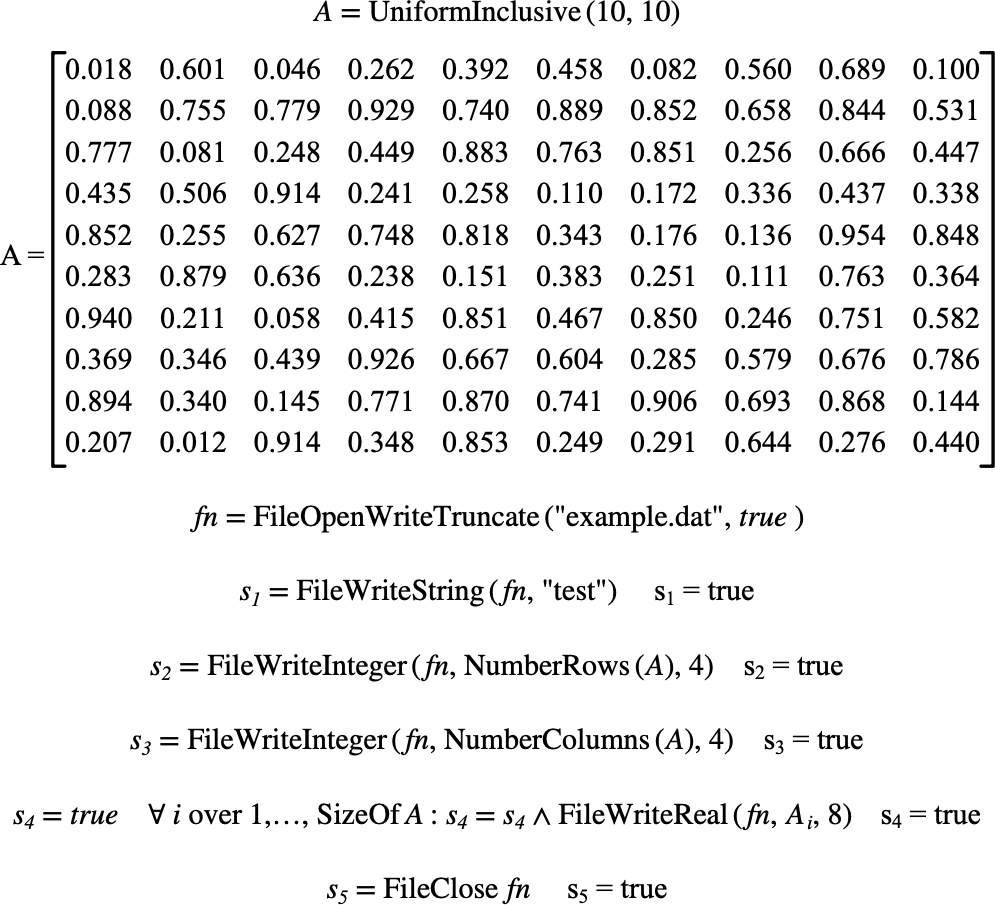# $$\text{FileOpenWriteTruncate}$$¶

You can use the $$\text{FileOpenWrite}$$ function to open a file for reading and writing, destroying existing content.

You can use the \fileopenwritetruncate backslash command to insert this function.

The following variants of this function are available:

• $$\text{integer } \text{FileOpenWriteTruncate} \left ( \text{<filename>} \right )$$

• $$\text{integer } \text{FileOpenWriteTruncate} \left ( \text{<filename>}, \text{<binary>} \right )$$

The $$\text{<filename>}$$ parameter is a text string encoded in a tuple holding the name of the file to be opened. If provided, the $$\text{<binary>}$$ parameter indicates if the file should be written in a binary or text format. If the $$\text{<binary>}$$ is not included, Aion will assume a text file. Note that the $$\text{<binary>}$$ parameter is only meaningful on Windows and modifies how the several control codes are interpreted.

On success, the $$\text{FileOpenWriteTruncate}$$ function returns a file number you can then use to interact with the file using any of the functions:

When you are done, you should close the file using the FileClose.

The Figure 136 shows how you can use the $$\text{FileOpenWriteTruncate}$$ function.Figure 136 Example Use Of The FileOpenWriteTruncate Function